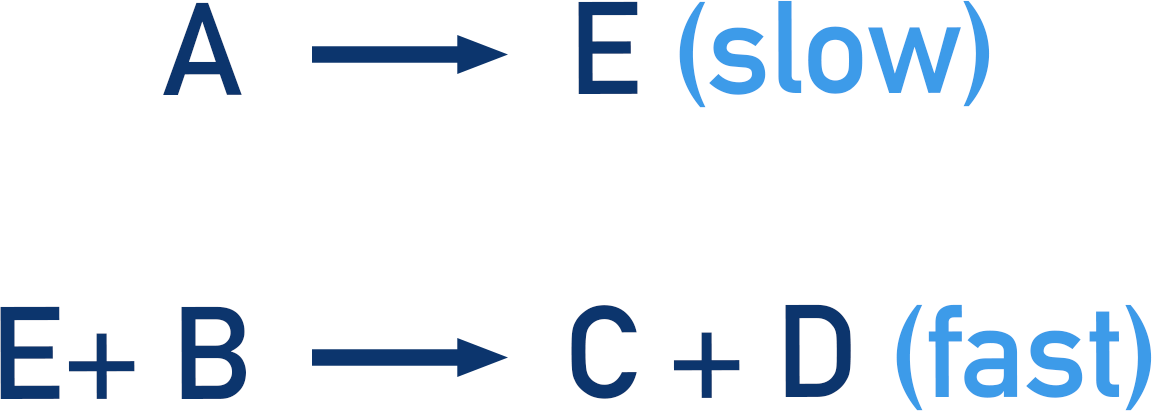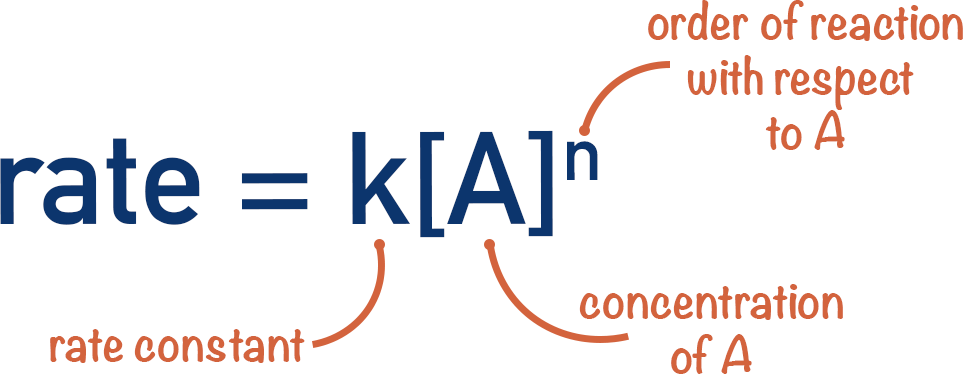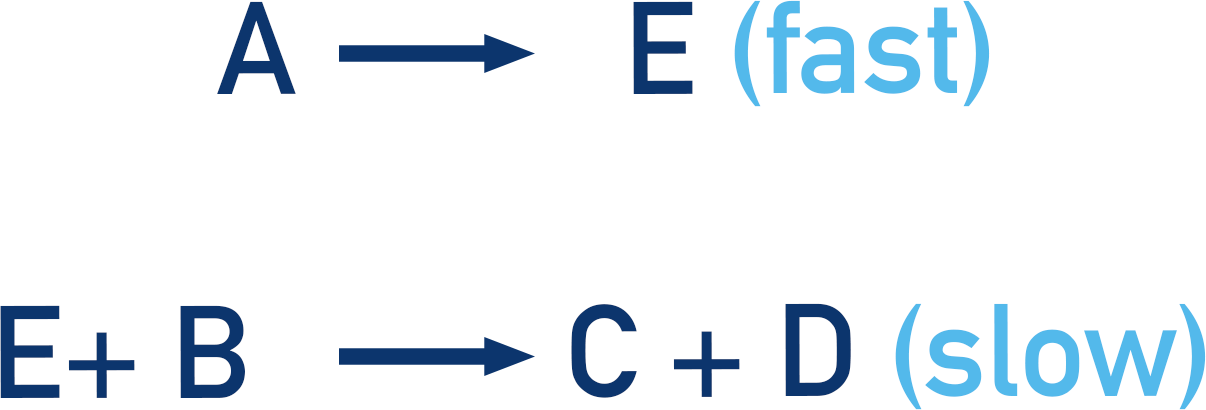Quick Notes Rate Determining Step and Mechanisms

• Reactions often happen in several steps (represented using mechanisms).
• The slowest step in a reaction determines the rate of the reaction and is called the rate determining step.
• The rate equation for a reaction can be used to help predict the mechanism of a reaction by showing the reactants involved in the rate determining step.

Full Notes Rate Determining Step and Mechanisms

Most reactions do not happen in one simple step, but in a series of steps. These steps do not always occur at the same speed. When looking at how fast a reaction can happen, it's the slowest step that determines the rate of the reaction.

Think of a marathon race – the total time of the marathon is actually how long it takes for the last person to cross the finish line, not the first. It does not matter how many runners finish in front of the last runner or how fast they run, the final runner across the line will always determine the overall time of the race.

For multi-step reactions, the slowest reaction step is controlling the overall speed of the reaction. It doesn’t matter how fast the other steps can happen, it’s all limited by the slowest step.

Imagine the reaction:Let’s say this reaction actually happens in the following way:There are two clear steps: the first involves just A, the second involves B and the product of the first step (E). The brackets tell us that the first step is the slowest. This means it must be the rate determining step. Think of the slowest marathon runner!

The rate of a reaction can be calculated mathematically using the rate equation for the reaction. As the rate determining step only involves the reactant A, the rate is going to be dependent on the concentration of A only. The amount of B will have no impact on the rate of the reaction because the step with B is fast. Think of the fast marathon runners – they have no impact on the overall time of the marathon.

This gives us the rate equation:Things get a little more complicated if the slow and fast steps were changed around.Now, the rate of the reaction is dependent on the second step. The second step involves B and E. E is not actually a reactant though, it’s formed from A.

The second step cannot happen until A has reacted to form E. The concentration of A will have an effect on how quickly E can be formed, and this has to be accounted for in the rate equation.Here it’s a little like a relay race. The slowest relay runner determines the length of the race for a team, but it relies on the relay baton from another runner in the team first!

Being able to predict possible mechanisms from a rate equation or predicting a rate equation from a mechanism is not easy. Practicing exam based questions and ensuring you are comfortable with the idea of the rate determining step is essential.